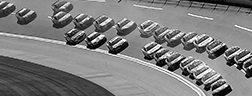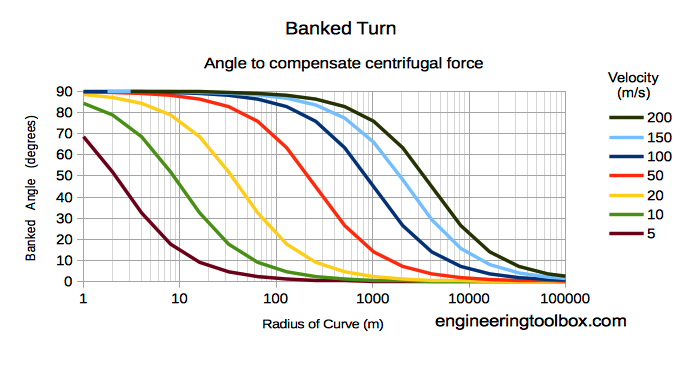Engineering ToolBox - Resources, Tools and Basic Information for Engineering and Design of Technical Applications!

# Banked Turn

## A turn or change of direction in which the vehicle banks or inclines, usually towards the inside of the turn.

For a vehicle moving in a circle - like a train or a car in a curve - the wheels on the vehicle produces a centripetal acceleration toward the center of the circle. The road or track experiences a centrifugal thrust that tries to move the road or track outwards.

The outwards thrust can be reduced by inclining the outside of the track. The inclined angle - or banked angle - is the angle at which a vehicle is inclined about its longitudinal axis with respect to its path.The banked angle can be calculated in radians as

Θrad = tan-1(v2 / (r ag))                    (1)

where

v = velocity (m/s)

r = radius of the curve (m)

ag = acceleration of gravity (9.91 m/s2

- or alternatively in degrees

Θdegrees = tan-1(v2 / (r ag)) (360 / 2 π)                 (1b)

### Example - A Train on a Railway Track in a Curve with Radius 1000 m with Speed 70 km/h

The required banked angle to avoid the centrifugal force can be calculated:

Θ = tan-1(((70 km/h) (1000 m/km) / (3600 s/h))2 / ((1000 m) (9.81 m/s2)))

= (0.0385 rad) (360 / 2 π)

= 2.2o

### Banked Turn Calculator

This calculator can be used to calculate the centripetal acceleration of the car and the banked angle.

The diagram below indicates velocity vs. curve radius and required banked turn to compensate centrifugal forces.## Related Topics

• ### Dynamics

Motion - velocity and acceleration, forces and torque.

## Related Documents

• ### Acceleration of Gravity and Newton's Second Law

Acceleration of gravity and Newton's Second Law - SI and Imperial units.
• ### Acceleration Units Converter

Converting between units of acceleration.
• ### Centripetal and Centrifugal Acceleration Force

Forces due to circular motion and centripetal / centrifugal acceleration.
• ### Formulas of Motion - Linear and Circular

Linear and angular (rotation) acceleration, velocity, speed and distance.
• ### Vehicles Traffic Flow and Density

Traffic flow and density as used in highway design.

## Engineering ToolBox - SketchUp Extension - Online 3D modeling!

Add standard and customized parametric components - like flange beams, lumbers, piping, stairs and more - to your Sketchup model with the Engineering ToolBox - SketchUp Extension - enabled for use with older versions of the amazing SketchUp Make and the newer "up to date" SketchUp Pro . Add the Engineering ToolBox extension to your SketchUp Make/Pro from the Extension Warehouse !

We don't collect information from our users. More about

## Citation

• The Engineering ToolBox (2012). Banked Turn. [online] Available at: https://www.engineeringtoolbox.com/banked-turn-d_1844.html [Accessed Day Month Year].

Modify the access date according your visit.

10.2.10